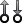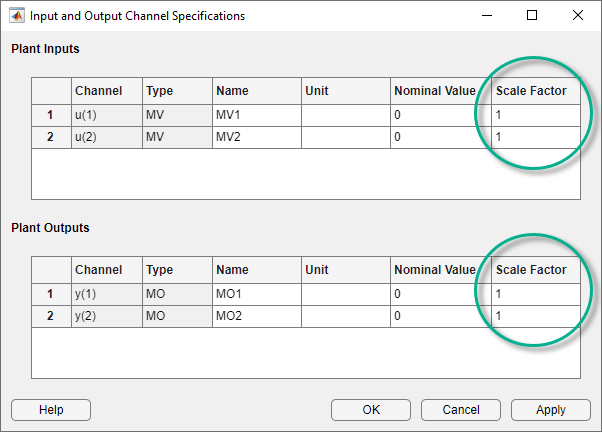Main Content

## Specify Scale Factors

Recommended practice includes specification of scale factors for each plant input and output variable, which is especially important when certain variables have much larger or smaller magnitudes than others.

The scale factor should equal (or approximate) the span of the variable. Span is the difference between its maximum and minimum value in engineering units, that is, the unit of measure specified in the plant model. Internally, MPC divides each plant input and output signal by its scale factor to generate dimensionless signals.

The potential benefits of scaling are as follows:

• Default MPC tuning weights work best when all signals are of order unity. Appropriate scale factors make the default weights a good starting point for controller tuning and refinement.

• When choosing cost function weights, you can focus on the relative priority of each term rather than a combination of priority and signal scale.

• Improved numerical conditioning. When values are scaled, round-off errors have less impact on calculations.

Once you have tuned the controller, changing a scale factor is likely to affect performance and the controller may need retuning. Best practice is to establish scale factors at the beginning of controller design and hold them constant thereafter.

You can define scale factors at the command line and using the MPC Designer app.

### Determine Scale Factors

To identify scale factors, estimate the span of each plant input and output variable in engineering units.

• If the signal has known bounds, use the difference between the upper and lower limit.

• If you do not know the signal bounds, consider running open-loop plant model simulations. You can vary the inputs over their likely ranges, and record output signal spans.

• If you have no idea, use the default scale factor (=1).

### Specify Scale Factors at Command Line

After you create the MPC controller object using the `mpc` command, set the scale factor property for each plant input and output variable.

For example, the following commands create a random plant, specify the signal types, and define a scale factor for each signal.

```% Random plant for illustrative purposes: 5 inputs, 3 outputs Plant = drss(4,3,5); Plant.InputName = {'MV1','UD1','MV2','UD2','MD'}; Plant.OutputName = {'UO','MO1','MO2'}; % Example signal spans Uspan = [2, 20, 0.1, 5, 2000]; Yspan = [0.01, 400, 75]; % Example signal type specifications iMV = [1 3]; iMD = 5; iUD = [2 4]; iDV = [iMD,iUD]; Plant = setmpcsignals(Plant,'MV',iMV,'MD',iMD,'UD',iUD, ... 'MO',[2 3],'UO',1); Plant.D(:,iMV) = 0; % MPC requires zero direct MV feed-through % Controller object creation. Ts = 0.3 for illustration. MPCobj = mpc(Plant,0.3); % Override default scale factors using specified spans for i = 1:2 MPCobj.MV(i).ScaleFactor = Uspan(iMV(i)); end % NOTE: DV sequence is MD followed by UD for i = 1:3 MPCobj.DV(i).ScaleFactor = Uspan(iDV(i)); end for i = 1:3 MPCobj.OV(i).ScaleFactor = Yspan(i); end ```

### Specify Scale Factors Using MPC Designer

After opening MPC Designer and defining the initial MPC structure, on the MPC Designer tab, click I/O Attributes.

In the Input and Output Channel Specifications dialog box, specify a Scale Factor for each input and output signal.To update the controller settings, click .

## Support

#### Implementing an Adaptive Cruise Controller with Simulink

Download technical paper